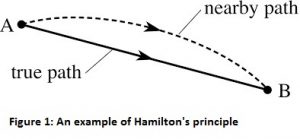Angus Alexander, University of Wollongong

The motivation for this project lies in physics. Physics is the study of matter, energy and the interaction between them. To study this interaction, we often want to develop equations of motion and solve them to develop a picture of how our system will behave. A common technique for doing this is to develop what is called an action principle. For more information on these see .

One of the earliest action principles, Fermat’s principle (also known as the principle of least time), was developed in the 17th century by French mathematician Pierre de Fermat and states that the path light travels between two points is the path which takes the least time. This principle can be used to describe the properties of light in many optical systems, such as reflection from mirrors.

Possibly the most well known action principle is Hamilton’s principle. Also known as the principle of least action, Hamilton’s principle was developed in the 19th century by Irish mathematician William Rowan Hamilton. The principle of least action states that the actual path travelled by a particle will be that which minimises the action, a specific function of the kinetic and potential energy. The principle of least action is fundamental in classical mechanics and equivalent to Newton’s third law of motion. Figure 1  below demonstrates the actual path taken by a particle in comparison to a path of greater action.In the 20th century physicist Richard Feynman applied this technique to quantum mechanics (the study of the behaviour of subatomic particles), however his approach is difficult to work with and often poorly defined. Feynman’s approach does not work when our particles approach the speed of light, as is the case in quantum relativistic mechanics. The behaviour of particles in this setting is often quite strange and difficult to determine.

Feynman created a pictorial representation of these, Feynman diagrams. Lines represent the trajectories of particles, with squiggly and straight lines depicting different types of particles, moving from left to right. The intersection of lines indicates particle interactions and emissions, the processes we wish to study.Figure 2  above shows an electron and positron pair annihilating and producing a photon that decays into a quark-antiquark pair, which radiates a gluon.

In the 1990s mathematician Alain Connes’ developed an action principle for relativistic quantum mechanics in an idealistic situation, however this principle does not apply to real physical problems. The goal of this project was to investigate how recent results by Bar and Strohmaier  could be combined with that of Connes’  to develop such a principal for more realistic models. The main achievement of this project was analysing such a principle in the simple case of a particle on a cylinder.

References

 Wolfgang Yourgrau and Stanley Mandelstam. Variational Principles in Dynamics and Quantum Theory, General Publishing Company, 1968.

 University of Reading (1996). Rays and Geometrical Optics. http://www.met.reading.ac.uk/pplato2/h-flap/phys6_2.html . Retrieved 20/2/18

 Wikipedia Foundation, Inc. (2018). Feynman Diagram. https://en.wikipedia.org/wiki/Feynman_diagram . Retrieved 20/2/18

 Alain Connes. Gravity coupled with matter and the foundation of non commutative geometry. Communications in Mathematical Physics, (1):155-156, 1996.

 Christian Bar and Alexander Strohmaier. An index theorem for Lorentian manifolds with compact spacelike Cauchy boundary. American Journal of Mathematics, 2015.

Angus Alexander was one of the recipients of a 2017/18 AMSI Vacation Research Scholarship.